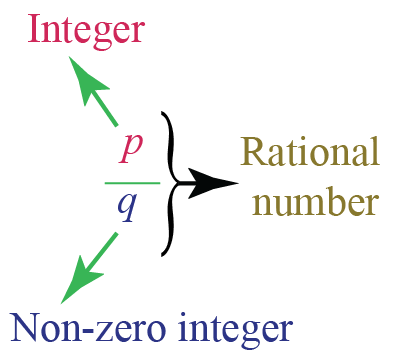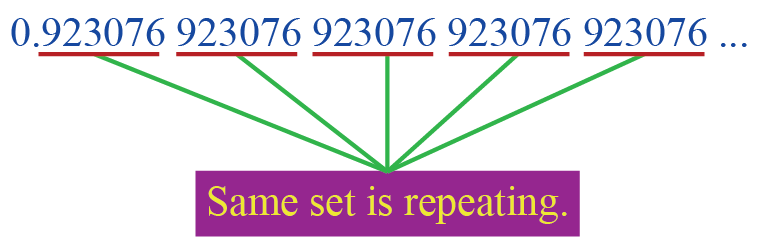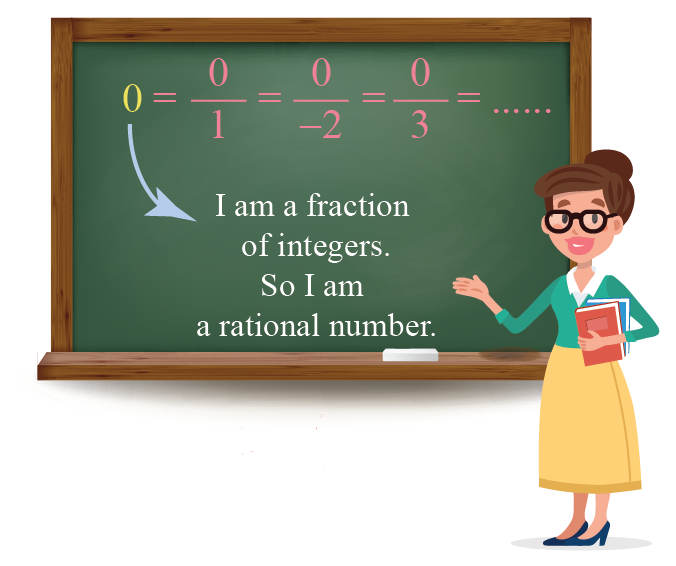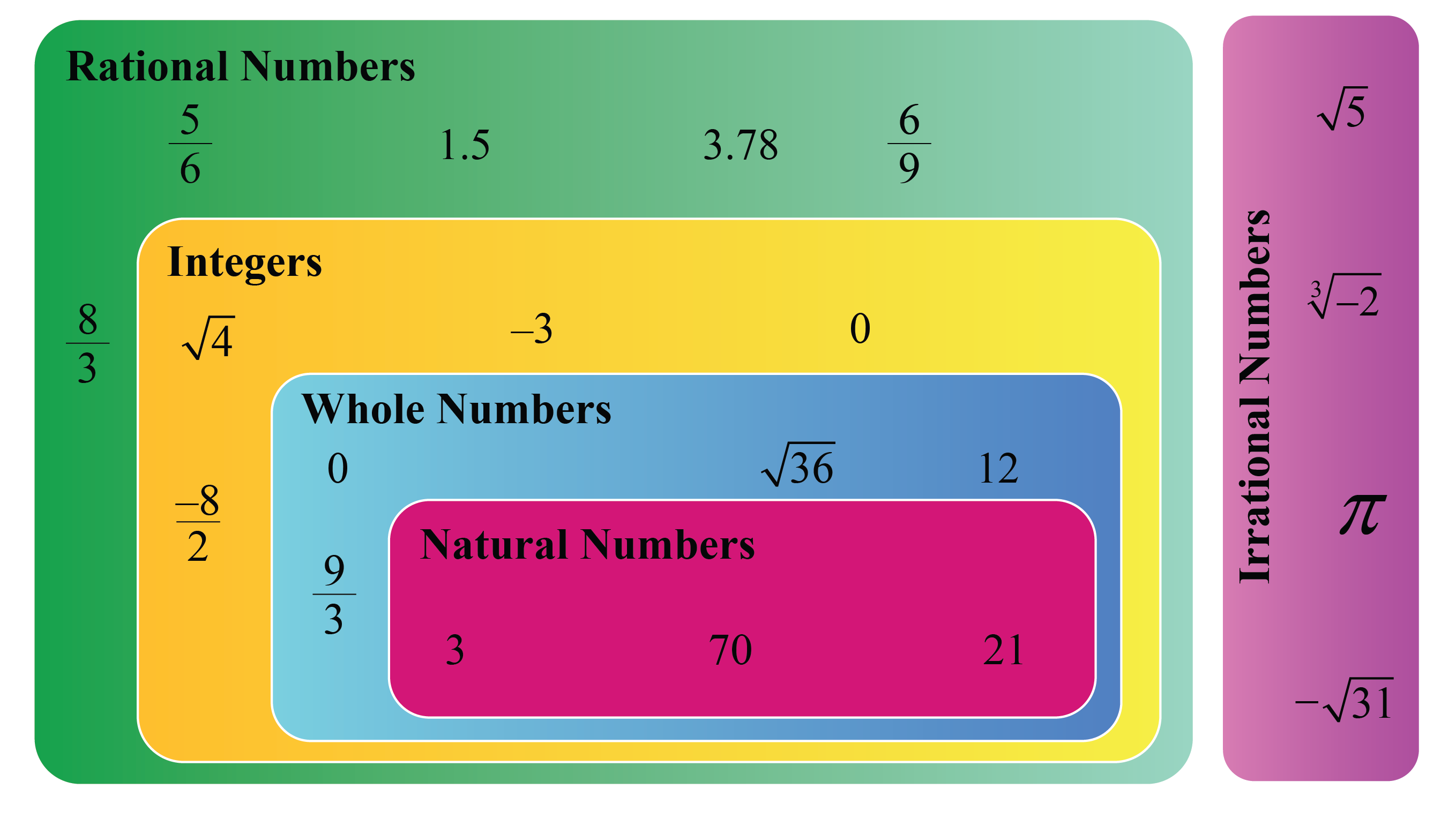# Rational Numbers

Rational Numbers
Go back to  'Numbers'

 1 Definition of Rational Numbers 2 Different Types of Rational Numbers 3 Important Notes on Rational Numbers 4 Arithmetic Operations on Rational Numbers 5 Irrational and Rational Numbers 6 Challenging Questions on Rational Numbers 7 Solved Examples on Rational Numbers 8 Important topics of Rational Numbers 9 Practice Questions on Rational Numbers 10 Maths Olympiad Sample Papers 11 Frequently Asked Questions (FAQs) 12 FREE Worksheets on Rational Numbers

We at Cuemath believe that Math is a life skill. Our Math Experts focus on the “Why” behind the “What.” Students can explore from a huge range of interactive worksheets, visuals, simulations, practice tests, and more to understand a concept in depth.

Book a FREE trial class today! and experience Cuemath's LIVE Online Class with your child.

## Definition of Rational Numbers

A rational number is a number that is of the form $$\dfrac{p}{q}$$ where:

• $$p$$ and $$q$$ are integers
• $$q \neq 0$$The set of rational numbers is denoted by $$Q$$.

### Examples of Rational Numbers

If a number can be expressed as a fraction where both the numerator and the denominator are integers, the number is a rational number.

Some examples of rational numbers are:

• $$\dfrac{1}{2}$$

• $$\dfrac{-3}{4}$$

• $$0.3$$ (or) $$\dfrac{3}{10}$$

• $$-0.7$$ (or) $$\dfrac{-7}{10}$$

• $$0.141414...$$ (or) $$\dfrac{14}{99}$$

## Different Types of Rational Numbers

There are different types of rational numbers.

We shouldn't assume that only fractions with integers are rational numbers.

The different types of rational numbers are:

• integers like $$-2, 0, 3,$$ etc.
• fractions whose numerator and denominators are integers like $$\dfrac{3}{7}$$, $$\dfrac{-6}{5}$$, etc.
• terminating decimals like $$0.35, 0.7116, 0.9768$$, etc.
• non-terminating decimals with some repeating patterns (after the decimal point) such as $$0.333..., 0.141414...$$, etc.

### How to Identify Rational Numbers?

In each of the above cases, the number can be expressed as a fraction of integers.

Hence, each of these numbers is a rational number.

To find whether a given number is a rational number, we can check whether it matches with any of these conditions.

Example:

Is $$0.923076923076923076923076923076...$$ a rational number?

Solution:

The given number has a set of decimals $$923076$$ which is repeating continuously.Thus, it is a rational number.

### Is 0 a Rational Number?

Yes, $$0$$ is a rational number as it can be written as a fraction of integers like $$\dfrac{0}{1}, \dfrac{0}{-2},...$$ etc.### List of Rational Numbers

From the above information, it is clear that there are an infinite number of rational numbers.

Hence, it is not possible to determine the list of rational numbers.

### Smallest Rational Number

Since we cannot determine the list of rational numbers, we cannot determine the smallest rational number.

### Adding and Subtracting Rational Numbers

The process of adding and subtracting rational numbers can be done in the same way as fractions.

To add or subtract any two rational numbers, we make their denominators the same and then add the numerators.

Example :

\begin{aligned}\dfrac{1}{2}- \dfrac{-2}{3} &= \dfrac{1}{2}- \left(-\dfrac{2}{3}\right)\\[0.3cm]&=\dfrac{1}{2} \times \dfrac{3}{3}+ \dfrac{2}{3}\times \dfrac{2}{2}\\[0.3cm] &= \dfrac{2}{6}+ \dfrac{4}{6}\\[0.3cm] &= \dfrac{6}{6}\\[0.3cm] &= \color{blue}{\boxed{\mathbf{1}}} \end{aligned}

### Multiplying and Dividing Rational Numbers

The process of multiplying and dividing rational numbers can be done in the same way as fractions.

To multiply any two rational numbers, we multiply their numerators and their denominators separately and simplify the resultant fraction.

Example:

$\dfrac{3}{5} \times \dfrac{-2}{7} = \dfrac{3 \times -2}{5 \times 7}= \color{blue}{\boxed{\mathbf{\dfrac{-6}{35}}} }$

To divide any two fractions, we multiply the first fraction (which is dividend) by the reciprocal of the second fraction (which is the divisor).

Example:

$\dfrac{3}{5} \div \dfrac{2}{7}=\dfrac{3}{5} \times \dfrac{7}{2}= \color{blue}{\boxed{\mathbf{\dfrac{21}{10}}}} \text{ (OR) } \color{blue}{\boxed{\mathbf{2 \dfrac{1}{10}}}}$

## Irrational and Rational Numbers

The numbers which are NOT rational numbers are called irrational numbers.

The set of irrational numbers is represented by $$I$$.

The differences between rational and irrational numbers are as follows:

Rational Numbers Irrational Numbers

These are numbers that can be expressed as fractions of integers.

Examples: $$0.75, \dfrac{-31}{5}$$, etc

These are numbers that CANNOT be expressed as fractions of integers.

Examples: $$\sqrt{5}, \sqrt{-2}$$, etc.

They can be terminating decimals. They are NEVER terminating decimals.

They can be non-terminating decimals with repetitive patterns of decimals.

Example: $$1.414 \, 414 \, 414 ...$$ has repeating patterns of decimals where $$414$$ is repeating.

They should be non-terminating decimals with NO repetitive patterns of decimals.

Example: $$\sqrt{5} = 2.236067977499789696409173....$$ has no repeating patterns of decimals

The set of rational numbers contains all natural numbers, all whole numbers, and all integers. The set of irrational numbers is a separate set and it does NOT contain any of the other sets of numbers.We can understand the formation of rational and irrational numbers using the following simulation:Challenging Questions
1. Is $$\sqrt{4}$$ rational or irrational?
2. Is $$\dfrac{\sqrt{7}}{2}$$ rational or irrational?
3. Is $$\pi$$ rational or irrational?
Hint: Note that the exact value of $$\pi$$ is NOT $$\dfrac{22}{7}$$. Its value is a decimal $$3.141592653589793238...$$, which has no repeating patterns of decimals.

Help your child score higher with Cuemath’s proprietary FREE Diagnostic Test. Get access to detailed reports, customized learning plans, and a FREE counseling session. Attempt the test now.

## Solved Examples

 Example 1

Identify the rational numbers among the following:

$\sqrt{4},\, \sqrt{3},\, \dfrac{\sqrt{5}}{2},\, \dfrac{-4}{5},\, \pi,\, 1.41421356237309504...$

Solution:

A rational number when simplified should either be a terminating decimal or a non-terminating decimal with repeating patterns of decimals.

Thus, the rational numbers among the given numbers are:

 $\mathbf{ \sqrt{4} \text{ and } \dfrac{-4}{5} }$
 Example 2

Find a rational number that lies between the following rational numbers:

$\dfrac{1}{2} \text{ and } \dfrac{2}{3}$

Solution:

We know that the average of any two numbers lies between the two numbers.

Let's find the average of the given two rational numbers.

\begin{aligned} \dfrac{ \dfrac{1}{2}+ \dfrac{2}{3}}{2} &= \dfrac{\dfrac{3}{6}+ \dfrac{4}{6}}{2}\\[0.3cm] &= \dfrac{ \left(\dfrac{7}{6} \right)}{2}\\[0.3cm] &= \dfrac{ \left(\dfrac{7}{6} \right)}{ \left(\dfrac{2}{1} \right)}\\[0.3cm] &= \dfrac{7}{6} \times \dfrac{1}{2}\\[0.3cm] &= \dfrac{7}{12} \end{aligned}

Thus, the rational number is:

 $\mathbf{\dfrac{7}{12}}$

CLUEless in Math? Check out how CUEMATH Teachers will explain Rational numbers to your kid using interactive simulations & worksheets so they never have to memorise anything in Math again!

Explore Cuemath Live, Interactive & Personalised Online Classes to make your kid a Math Expert. Book a FREE trial class today!

## Practice Questions

Here are few activities for you to practice. Select/Type your answer and click the "Check Answer" button to see the result.

## Given below are the list of topics that are closely connected to Rational Numbers. These topics will also give you a glimpse of how such concepts are covered in Cuemath.

IMO (International Maths Olympiad) is a competitive exam in Mathematics conducted annually for school students. It encourages children to develop their math solving skills from a competition perspective.

## 1. What is a rational number? Give an example.

Any number that is of the form $$\dfrac{p}{q}$$ where both $$p$$ and $$q$$ are integers is a rational number.

Some examples of rational numbers are:

• $$\dfrac{1}{2}$$

• $$\dfrac{-3}{4}$$

• $$0.3$$ (or) $$\dfrac{3}{10}$$

• $$-0.7$$ (or) $$\dfrac{-7}{10}$$

• $$0.141414...$$ (or) $$\dfrac{14}{99}$$

## 2. Is 3.14 a rational number?

Yes, $$3.14$$ is a rational number as it is a terminating decimal.

But note that $$\pi$$ is NOT a rational number because the exact value of $$\pi$$ is NOT $$\dfrac{22}{7}$$

Its value is a decimal $$3.141592653589793238...$$ which has no repeating patterns of decimals.

## Is 7 a rational number?

Yes, $$7$$ is a rational number as it can be expressed as a fraction of integers $$\dfrac{7}{1}$$

## 3. How can you identify a rational number?

To identify whether a given number is rational, just convert it into decimal form.

If the decimal is either terminating or non-terminating with repeating patterns of decimal, the number is rational.

Otherwise, the number is irrational.

## 4. Is 0 an irrational number?

No, $$0$$ is a rational number as it can be written as a fraction of integers like $$\dfrac{0}{1}, \dfrac{0}{-2},...$$ etc.

Numbers and Number Systems
grade 9 | Questions Set 2
Numbers and Number Systems
Numbers and Number Systems